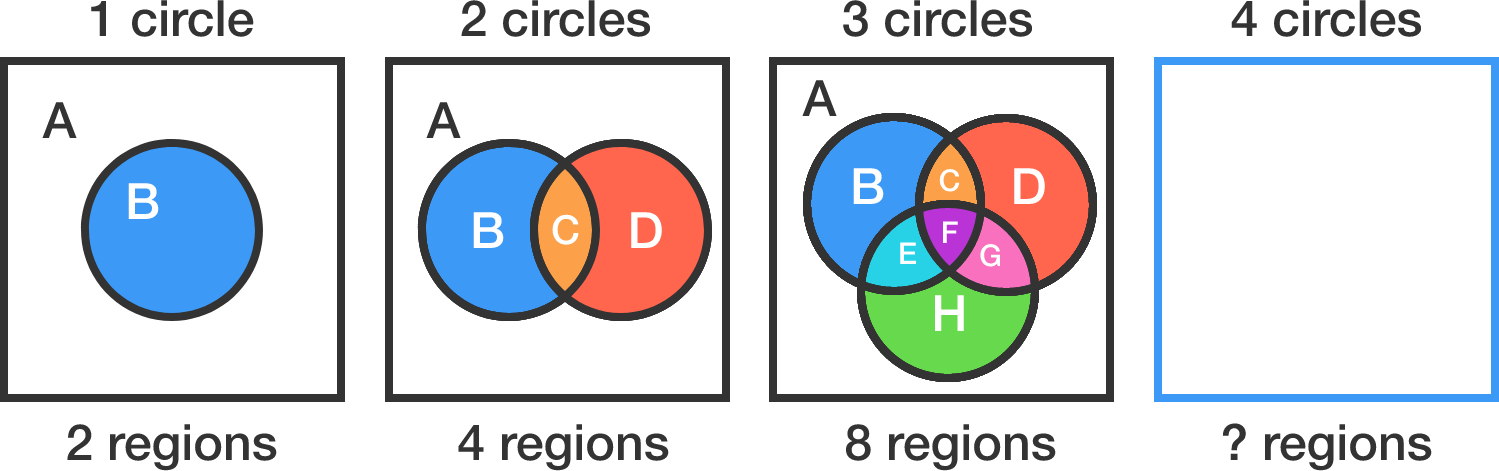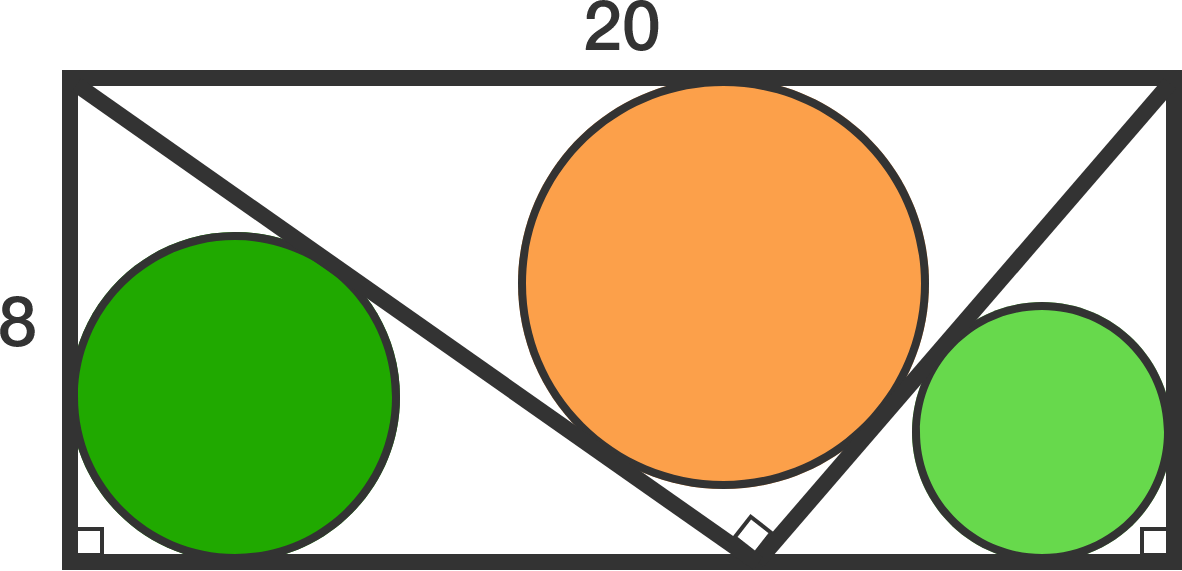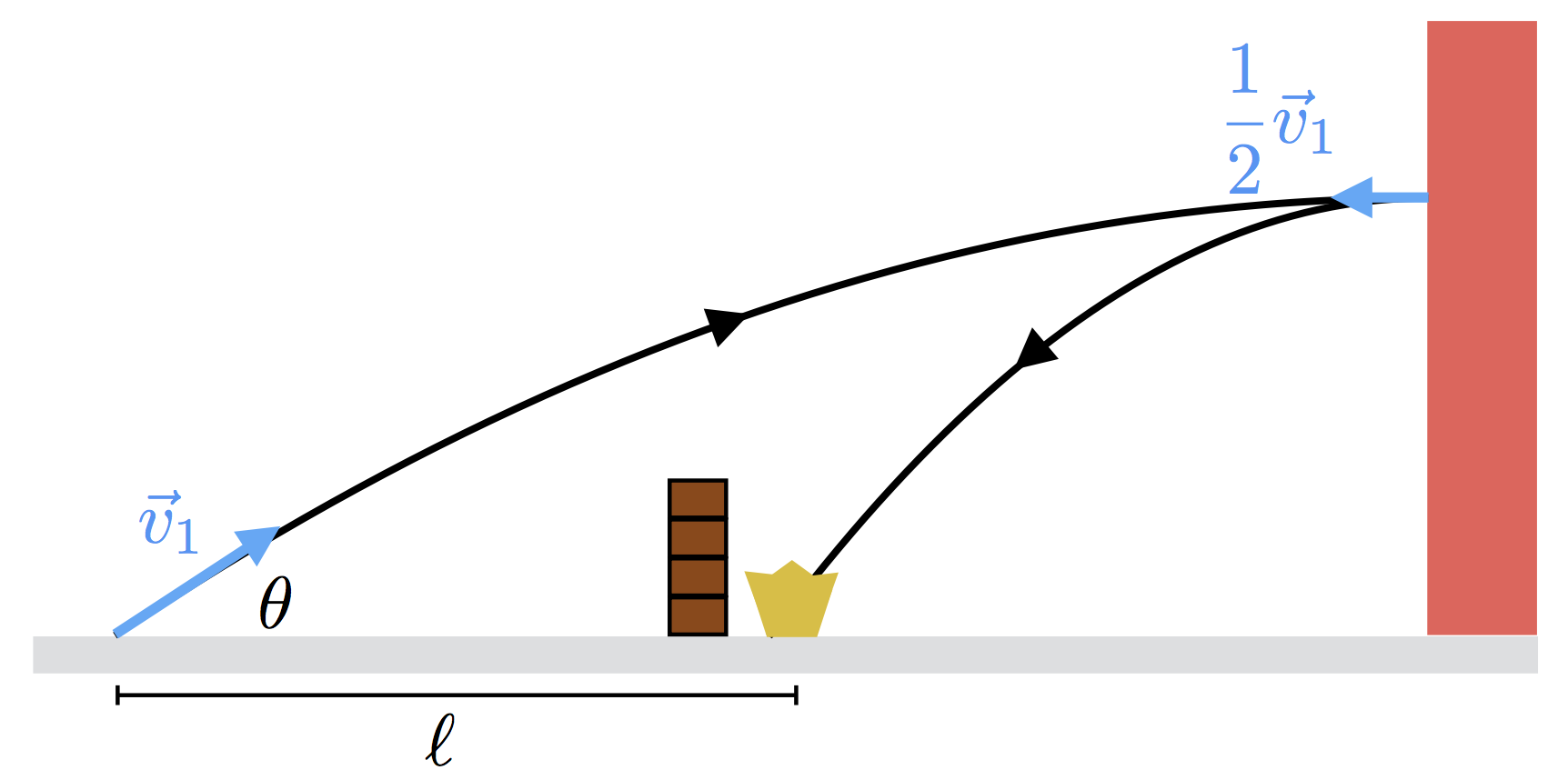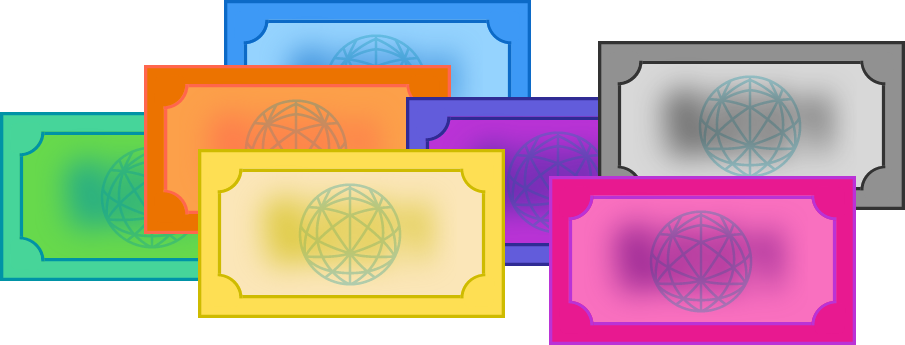# Problems of the Week

Contribute a problem

# 2017-08-28 Intermediate

1 circle can split the plane into at most 2 regions.
2 circles can split the plane into at most 4 regions.
3 circles can split the plane into at most 8 regions.
4 circles can split the plane into at most $\text{\_\_\_\_\_\_}$ regions.In a game of tennis, does having "deuce" (as compared to a simple "first to 4 points" system) help, hurt, or not affect the better player?


Details and Assumptions:

• The "better player" wins each point with fixed probability $p > 0.5.$
• In "deuce" rules, if the game reaches 3-3, the winner is the first person to subsequently lead by 2 points (colloquially, "win by 2").

A $20\times8$ rectangle is partitioned into three right triangles, and a circle is inscribed in each triangle.

Find the sum of the radii of these 3 circles.There's a war on the playground, and you're the charismatic leader of the Greasers. The Socs, your sworn enemies, have fashioned themselves a defensive fort, behind which they're hiding their prized possession, the crown.

The only way to win the war is by making a direct hit on the crown with a tennis ball. Blocked from a direct hit by the fort, the Greasers have to shoot it off the wall behind the fort and bank it into the crown.As shown in the diagram, the Greasers launch a tennis ball with speed $v_1 = \SI[per-mode=symbol]{20}{\meter\per\second}$ at an angle of $\theta=\SI{\frac{\pi}6}{\radian}$ with the horizontal. At the peak of its trajectory, the ball hits the wall and reflects with speed $\frac12 v_1.$

If the ball hits the crown as shown in the diagram, calculate the distance $\ell$ (in $\si{\meter}$) between the launch point and the crown.

Assume that $g = \SI[per-mode=symbol]{9.81}{\meter\per\second\squared}.$

I have 7 distinct dollar bills, each of which is a distinct integer denomination of $50 or less. Using these bills, I can buy anything that costs an integer amount between$1 and \$60 (inclusive) in exact change.

What is the least possible value of the largest denomination of these 7 bills (in dollars)?×